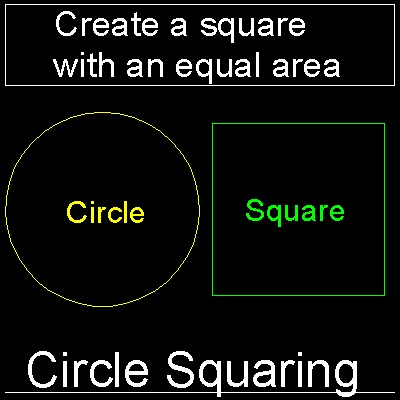History of the Problems
Go to   Fun_Math Content Table   Three Famous Problems

### History of the problem

#### Problem Definition:To construct a square which has the same area as the given circle.

This is equivalent to construct a line segment of length p or its square root.

******** circle_squaring_problem.dwg *********

#### Origin of the Problem :

The circle is the simplest , and hence the first curve men has created. So naturally from the earliest days

in history, men have had a keen interest in the area and circumference of this curve.

#### Rule of the Game ( Platonian Rule ) :

##### Tools allowed
the compasses and unmarked straight-edge
##### Permitted use of these 2 tools
1. The drawing of a straight line of indefinite length through two given points.

2. The construction of a circle with center at a given point and passing through a second given point.

#### Proof of impossibility:

In 1880 Ferndinand von Lindemann (1852 - 1939) proved that p is transcendental, that it is not the root of any polynomial equation

with rational coefficients. Thus he proved that it is not possible to square the circle with ruler and compass.